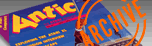`ANTIC VOL. 2, NO. 7 / OCTOBER 1983`

# COMPUTER QUIZ

by GUY HURT

1. Subroutines:
(a) are program segments that may be called from places of a program
(b) are useful to avoid duplicating programming efforts
(c) can help organize organize programs into logical blocks of code
(d) all of the above
(e) none of the above

2. What does the following program logic do? T = X:X == Y:Y=T
(a) increases the value of two variables
(b) increases the value of three variables
(c) alters the contents of two variables
(d) exchanges the contents of two variables
(e) non of the above

3. PEEK, the BASIC command on the ATARI, means to:
(a) alter the contents of a memory location
(b) look at the contents of a memory location
(c) erase the contents of a memory location
(d) exchange the contents of two memory locations
(e) none of the above

4. An acoustic coupler is an example of a:
(a) direct data service
(b) time-shared terminal
(c) modem
(d) multiplexor
(e) none of the above

5. The amount of required RAM for Graphics Mode 8 is:
(a) 5900 bytes
(b) 6900 bytes
(c) 7900 bytes
(d) 8900 bytes
(e) none of these

6. Memory location 707 contains a number representing a:
(a) color
(b) position
(c) status
(d) size
(e) none of these

7. The screen editor is symbolized by whht letter?
(a) S
(b) C
(c) E
(d) D
(e) none of these

8. The Atari 810 disk drive averages ? bits per second data transfer rate.
(a) 5,000
(b) 6, 000
(c) 7,000
(d) 8,000
(e) none of these

9. The internal code of the last key pressed may be found in memory location:
(a) \$IFC
(b) \$2FC
(c) \$3FC
(d) \$3CF
(e) none of these

10. SECONDS maybe calculated as:
(a) PEEK(19)*256+PEEK(20)
(b) PEEK(18)*256+PEEK(19)
(c) (PEEK(19)*256+PEEK(20))/3600
(d) (PEEK(19)*256+PEEK(20))/60
(e) none of the above

11. Examine the memory diagram below.
----------------
----FREE RAM----
--DISPLAY LIST--
??????????????
--TEXT WINDOW--
----------------

The item missing is:
(a) TOKENIZED BASIC
(b) SCREEN RAM
(c) STACK
(d) PAGE SIX
(e) none of these

12. Examine the following program:
5 GR.2: PLOT 0,0:DRAWTO 0,5:DRAWTO 5,5:DRAWTO 5,0:DRAWTO 0,0:END
The output from this program is:
(a) a square
(b) a rectangle
(c) a triangle
(d) a parallelogram
(e) nothing

13. D. is an abbrevation for:
(a) DIM
(b) DOS
(c) DATA
(d) DEG
(e) none of these

14. The floating point number 7.OE-21 is the same as:
(a) .00000000000000000007
(b) .000000000000000000007
(c) .0000000000000000000007
(d) .7x1000000000000000000000
(e) none of these

15. What is an example of ROM?
(a) an assembler routine
(b) a BASIC game program on disk
(c) the CENTIPEDE game cartridge
(d) a user-written BASIC program UTILITY
(e) none of the above

Answers for Quiz #2: (1. d) (2. d) (3. b) (4. c) (5. c) (6. a) (7. c) (8. b) (9. b) (10. d) (11. b) (12. e) (13. c) (14. b) (15. c)